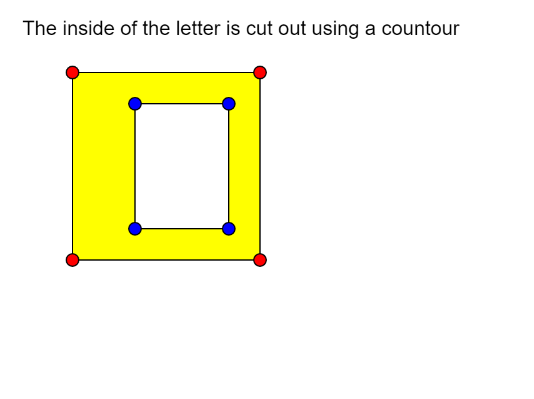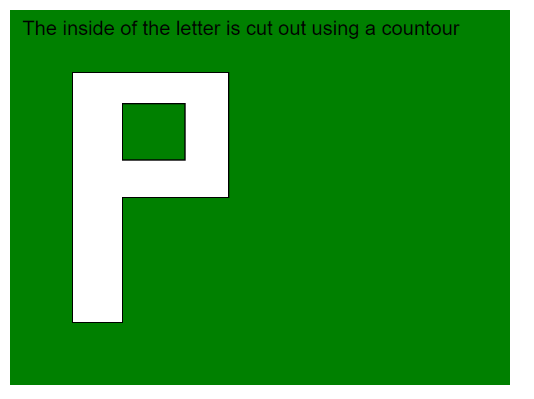# p5.js | beginContour() Function

The beginContour() function in p5.js is used to create negative shapes in other shapes, that is, it can be used to remove a portion of a shape with the given vertices. This function starts the recording of the shape that has to be removed. It is used with the endContour() function that stops the recording of the vertices.

The vertices of the interior shape have to be defined in the opposite direction to that of the exterior one. If the exterior shape has the vertices defined in the clockwise order, then the interior shape has to be defined in the counter-clockwise direction.

This function can only be used inside the beginShape() or endShape() function. Transformations like translate(), rotate() and scale() do not work with shapes and contours.

Syntax:

`beginContour()`

Parameters: This function accepts no parameters.

The program below illustrates the beginContour() function in p5.js:

Example 1:

 `function` `setup() { ` `  ``createCanvas(400, 300); ` `  ``textSize(16); ` `} ` ` `  `function` `draw() { ` `  ``clear(); ` `  ``fill(``"black"``); ` `  ``text(``"The inside of the letter is cut"``+ ` `       ``" out using a countour"``, 10, 20); ` `  ``fill(``"yellow"``); ` ` `  `  ``// Starting the shape using beginShape() ` `  ``beginShape(); ` ` `  `  ``// Specifying all the vertices ` `  ``// of the exterior shape ` `  ``vertex(50, 50); ` `  ``vertex(200, 50); ` `  ``vertex(200, 200); ` `  ``vertex(50, 200); ` ` `  `  ``// Starting a contour ` `  ``beginContour(); ` ` `  `  ``// Specifying all the vertices ` `  ``// of the interior shape ` `  ``// in counter-clockwise order ` `  ``vertex(100, 175); ` `  ``vertex(175, 175); ` `  ``vertex(175, 75); ` `  ``vertex(100, 75); ` ` `  `  ``// Ending the contour ` `  ``endContour(); ` ` `  `  ``// Ending the shape ` `  ``endShape(CLOSE); ` ` `  `  ``// Draw Circles for demonstration ` `  ``// Red ones for exterior shape ` `  ``fill(``"red"``); ` `  ``circle(50, 50, 10); ` `  ``circle(200, 50, 10); ` `  ``circle(200, 200, 10); ` `  ``circle(50, 200, 10); ` ` `  `  ``fill(``"blue"``); ` `  ``// Blue ones for interior shape ` `  ``circle(100, 175, 10); ` `  ``circle(175, 175, 10); ` `  ``circle(175, 75, 10); ` `  ``circle(100, 75, 10); ` `} `

Output:Example 2:

 `function` `setup() { ` `  ``createCanvas(400, 300); ` `  ``textSize(16); ` `} ` ` `  `function` `draw() { ` `  ``clear(); ` `  ``background(``"green"``); ` `  ``text(``"The inside of the letter is cut out"``+ ` `       ``" using a countour"``, 10, 20); ` ` `  `  ``// Starting the shape using beginShape() ` `  ``beginShape(); ` ` `  `  ``// Specifying all the vertices ` `  ``// of the exterior shape ` `  ``vertex(50, 250); ` `  ``vertex(50, 50); ` `  ``vertex(175, 50); ` `  ``vertex(175, 150); ` `  ``vertex(90, 150); ` `  ``vertex(90, 250); ` ` `  `  ``// Starting a contour ` `  ``beginContour(); ` ` `  `  ``// Specifying all the vertices ` `  ``// of the interior shape ` `  ``// in counter-clockwise order ` `  ``vertex(90, 120); ` `  ``vertex(140, 120); ` `  ``vertex(140, 75); ` `  ``vertex(90, 75); ` ` `  `  ``// Ending the contour ` `  ``endContour(); ` ` `  `  ``// Ending the shape ` `  ``endShape(CLOSE); ` `} `

Output:Online editor: https://editor.p5js.org/
Environment Setup: https://www.geeksforgeeks.org/p5-js-soundfile-object-installation-and-methods/My Personal Notes arrow_drop_upIm listening

If you like GeeksforGeeks and would like to contribute, you can also write an article using contribute.geeksforgeeks.org or mail your article to contribute@geeksforgeeks.org. See your article appearing on the GeeksforGeeks main page and help other Geeks.

Please Improve this article if you find anything incorrect by clicking on the "Improve Article" button below.

Article Tags :

Be the First to upvote.

Please write to us at contribute@geeksforgeeks.org to report any issue with the above content.